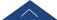﻿ 20200622 郭龙 Newton Polytopes of Polynomials in Algebraic Combinatorics-湖南大学数学学院

# 20200622 郭龙 Newton Polytopes of Polynomials in Algebraic Combinatorics

『组合数学』学术报告（五）

several important families of polynomials in algebraic
combinatorics, including for example Schubert polynomials,
Grothendieck polynomials, key polynomials. We develop
a combinatorial algorithm to generate the vertices of
the Newton polytopes of Schubert and key polynomials.
As an application, we show that the vertices of the Newton polytope of a key polynomial can be generated by permutations in a  Bruhat order interval, confirming a conjecture by Monical, Tokcan and Yong. This work is joint with Neil J.Y. Fan.